# Ratio Worksheet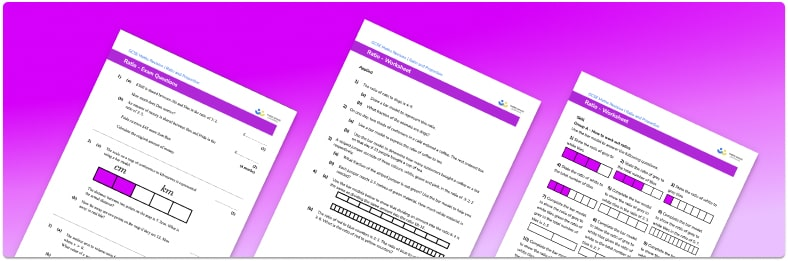• This field is for validation purposes and should be left unchanged.

You can unsubscribe at any time (each email we send will contain an easy way to unsubscribe). To find out more about how we use your data, see our privacy policy.

• Section 1 of the ratio worksheet contains 36 skills-based ratio questions, in 3 groups to support differentiation
• Section 2 contains 4 applied ratio questions with a mix of worded problems and deeper problem solving questions
• Section 3 contains 4 foundation and higher level GCSE exam style ratio questions
• Answers and a mark scheme for all ratio questions are provided
• Questions follow variation theory with plenty of opportunities for students to work independently at their own level
• All questions created by fully qualified expert secondary maths teachers
• Suitable for GCSE maths revision for AQA, OCR and Edexcel exam boards

### Ratio at a glance

A ratio compares how much there is of one thing in relation to another. For example if the ratio of the number of dogs to the number of cats is 2:3 then for every 2 dogs there are 3 cats.

Ratios can often be simplified by dividing each part by a common factor. Writing ratios in their simplest form makes it easier to visualise the relationship between the quantities in the ratios. It also makes it easier to use ratios in other contexts.

Ratio word problems may ask us to write a ratio, simplify a ratio, divide a quantity into a ratio or use a ratio to find quantities. We can use a bar model to represent a given ratio and this can help us visualise the ratio problem more easily.

Looking forward, students can then progress to additional ratio and proportion worksheets, for example aor a direct proportion worksheet.

For more teaching and learning support on Ratio and Proportion our GCSE maths lessons provide step by step support for all GCSE maths concepts.

## Related worksheets

Ratio To Fraction Worksheet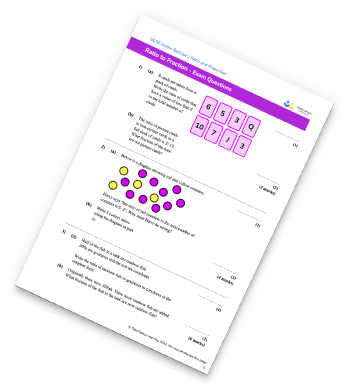Simplifying Ratios Worksheet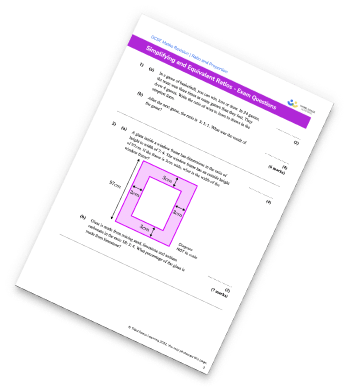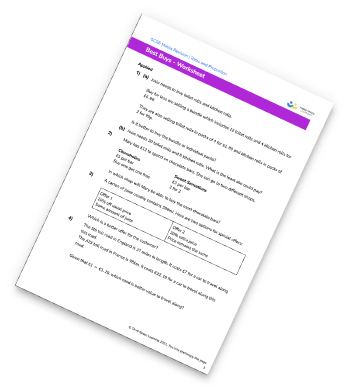Proportion Worksheet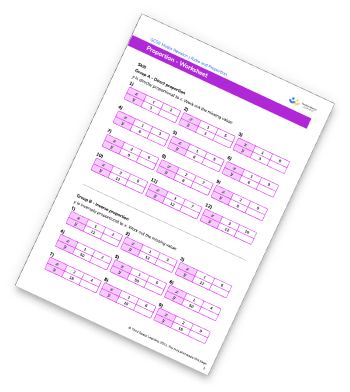## Do you have KS4 students who need more focused attention to succeed at GCSE?There will be students in your class who require individual attention to help them succeed in their maths GCSEs. In a class of 30, it’s not always easy to provide.

Help your students feel confident with exam-style questions and the strategies they’ll need to answer them correctly with our dedicated GCSE maths revision programme.

Lessons are selected to provide support where each student needs it most, and specially-trained GCSE maths tutors adapt the pitch and pace of each lesson. This ensures a personalised revision programme that raises grades and boosts confidence.

Find out more